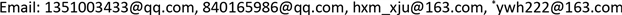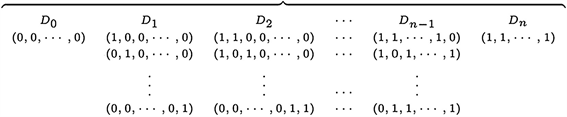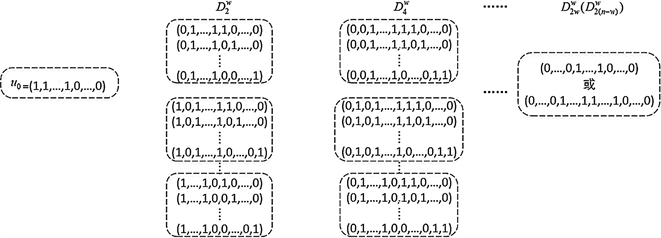﻿ 关于A(n,d,w)的一个注记 A Note on A(n,d,w)

Vol. 10  No. 03 ( 2021 ), Article ID: 41236 , 7 pages
10.12677/AAM.2021.103081A Note on $A\left(n,d,w\right)$

Yongfang Kou, Mengxin Lv, Xiaomin Hu, Weihua Yang*

School of Mathematics, Taiyuan University of Technology, Jinzhong ShanxiReceived: Feb. 23rd, 2021; accepted: Mar. 19th, 2021; published: Mar. 26th, 2021ABSTRACT

A basic problem in coding theory is to find the value of $A\left(n,d,w\right)$, that is the size of the maximum n-length binary constant weight code with the minimum Hamming distance d. However, it can be regarded as the size of the maximum independent set of ${Q}_{n}^{\left(d-1,w\right)}$ which is a subgraph of $d-{1}^{th}$ power of n-dimensional hypercube induced by all vertices with constant weight w. To explore the maximum independent set of ${Q}_{n}^{\left(d,w\right)}$, this paper gives the definition of ${Q}_{n}^{\left(d,w\right)}$ for the first time. Furthermore, some basic properties of the graph are studied and the main results are obtained as follows: ${Q}_{n}^{\left(d,w\right)}$ is ${\sum }_{i=1}^{⌊d/2⌋}\left(\begin{array}{c}w\\ w-i\end{array}\right)\left(\begin{array}{c}n-w\\ i\end{array}\right)$ -regular. ${Q}_{n}^{\left(d,w\right)}$ is vertex transitive. For $2\le d\le 3$, if $w\ge ⌈n/2⌉$, then $\omega \left({Q}_{n}^{\left(d,w\right)}\right)=w+1$ ; if $w<⌈n/2⌉$, then $\omega \left({Q}_{n}^{\left(d,w\right)}\right)=n-w+1$. For $3\le d\le 4$,$A\left(n,d,w\right)=\alpha \left({Q}_{n}^{\left(d-1,w\right)}\right)\le \frac{\left(\begin{array}{c}n\\ w\end{array}\right)}{w+1}$ or $\frac{\left(\begin{array}{c}n\\ w\end{array}\right)}{n-w+1}$.

Keywords:Hypercube, Maximum Independent Set, Coding Theory, Constant Weight Code1. 引言

n维二元向量空间 ${Z}_{2}^{n}={Z}_{2}×{Z}_{2}×\cdots ×{Z}_{2}$，可以将 ${Z}_{2}^{n}$ 中的每个n维二元向量看作是一个n长二元码字，码字构成的集合称为码集。码字x中非零码元的个数为码字x的Hamming重量，简称码重，记为 $wt\left(x\right)$。1950年，Hamming   定义两个码字 $x,y$ 间的Hamming距离为其不同码元的位数，记为 ${d}_{H}\left(x,y\right)$，即 ${d}_{H}\left(x,y\right)={\sum }_{i=1}^{n}|{x}_{i}-{y}_{i}|$$i\in \left[1,n\right]$

$A\left(n,d,w\right)$ 的上界做出了极大的改进，并证明了若d为偶数，则 $A\left(n,d,w\right)\le \frac{\left(\begin{array}{c}n\\ w-1\end{array}\right)}{w}$。此后，学者们继续利用半正定规划、Terwilliger代数等方法对 $A\left(n,d,w\right)$ 的上下界进行讨论，但大多都是对d取6，8，10，12时 $A\left(n,d,w\right)$ 的上下界有了一定的改进   。

2. 预备知识

$G=\left(V\left(G\right),E\left(G\right)\right)$ 为简单无向图，其中 $V\left(G\right)$ 是图G的顶点集， $E\left(G\right)$ 是图G的边集。任取 $v\in V\left(G\right)$，G中与点v关联的边的数目称为点v的度，记为 ${d}_{G}\left(v\right)$。若图G中所有点的度都为k，则称图G是k-正则的。令M是 $V\left(G\right)$ 的一个子集，若M中任意两个顶点在图G中均不相邻，则称M为图G的独立集。若图G中不包含满足 $|{M}^{*}|>|M|$ 的独立集 ${M}^{*}$，则称M为图G的最大独立集。图G的最大独立集的顶点数称为图G的独立数，记为 $\alpha \left(G\right)$。反之，若M中任意两个顶点在图G中均相邻，则称M为图G的团。若图G中不包含满足 $|{M}^{*}|>|M|$ 的团 ${M}^{*}$，则称M为图G的最大团，最大团的顶点数称为图G的团数，记为 $\omega \left(G\right)$

3. ${Q}_{n}^{\left(d,w\right)}$ 的基本性质Figure 1. The distance division graph of ${Q}_{n}^{d}$

${x}_{1}={x}_{2}=\cdots ={x}_{k}={x}_{k+1}=\cdots ={x}_{w}=1$${x}_{w+1}=\cdots ={x}_{n}=0$

${y}_{1}={y}_{2}=\cdots ={y}_{k}=1$${y}_{k+1}=\cdots ={y}_{w}=0$${y}_{w+1}=\cdots ={y}_{2w-k}=1$${y}_{2w-k+1}=\cdots ={y}_{n}=0$

$f=\left(\begin{array}{ccc}1& 2& \cdots \\ 1& 2& \cdots \end{array}\text{\hspace{0.17em}}\text{\hspace{0.17em}}\text{\hspace{0.17em}}\begin{array}{ccc}k& k+1& k+2\\ k& w+1& w+2\end{array}\text{\hspace{0.17em}}\text{\hspace{0.17em}}\text{\hspace{0.17em}}\begin{array}{ccc}\cdots & w& w+1\\ \cdots & 2w-k& k+1\end{array}\text{\hspace{0.17em}}\text{\hspace{0.17em}}\text{\hspace{0.17em}}\begin{array}{ccc}\cdots & 2w-k& 2w-k+1\\ \cdots & w& 2w-k+1\end{array}\text{\hspace{0.17em}}\text{\hspace{0.17em}}\text{\hspace{0.17em}}\begin{array}{cc}\cdots & n\\ \cdots & n\end{array}\right)$

1) $\phi$ 为双射。

$\phi$ 为单射：任取 $u,v\in V\left({Q}_{n}^{\left(d,w\right)}\right)$，若 $u\ne v$，则一定存在 $i\in \left\{1,2,\cdots ,n\right\}$${u}_{i}\ne {v}_{i}$。而对于 $i\in \left\{1,2,\cdots ,n\right\}$ 存在 $j\in \left\{1,2,\cdots ,n\right\}$ 使得 $f\left(j\right)=i$，故 ${u}_{f\left(j\right)}\ne {v}_{f\left(j\right)}$，即 $\phi \left(u\right)\ne \phi \left(v\right)$，故 $\phi$ 为单射。

$\phi$ 为满射：对于任意的 $u=\left({u}_{1},{u}_{2},\cdots ,{u}_{n}\right)\in V\left({Q}_{n}^{\left(d,w\right)}\right)$，存在 $v=\left({v}_{1},{v}_{2},\cdots ,{v}_{n}\right)\in V\left({Q}_{n}^{\left(d,w\right)}\right)$，其中

${v}_{i}={u}_{i}$ ( $1\le i\le k$$2w-k+1\le i\le n$ )，

${v}_{k+j}={u}_{w+j}\left(1\le j\le w-k\right)$

${v}_{w+j}={u}_{k+j}\left(1\le j\le w-k\right)$

2) 对于任意的 $u,v\in V\left({Q}_{n}^{\left(d,w\right)}\right)$$u~v$ 当且仅当 $\phi \left(u\right)~\phi \left(v\right)$

$\phi$ 和f定义可知， ${d}_{H}\left(\phi \left(u\right),\phi \left(v\right)\right)={\sum }_{i=1}^{n}|{u}_{f\left(i\right)}-{v}_{f\left(i\right)}|={\sum }_{i=1}^{n}|{u}_{i}-{v}_{i}|={d}_{H}\left(u,v\right)$，故 $u~v$ 当且仅当 $\phi \left(u\right)~\phi \left(v\right)$

$\omega \left({Q}_{n}^{\left(d,w\right)}\right)=\left\{\begin{array}{l}w+1\text{\hspace{0.17em}}\left(w\ge ⌈n/2⌉\right)\\ n-w+1\text{\hspace{0.17em}}\left(w<⌈n/2⌉\right)\end{array}$Figure 2. The distance division graph of ${Q}_{n}^{\left(d,w\right)}$

${Q}_{n}^{\left(d,w\right)}$ 的点传递性，我们只需考虑其包含点 ${u}^{0}$ 的最大团即可得 ${Q}_{n}^{\left(d,w\right)}$ 的团数。求 ${Q}_{n}^{\left(2,w\right)}$${Q}_{n}^{\left(3,w\right)}$ 最大团的方法完全一样，下面我们只给出 ${Q}_{n}^{\left(2,w\right)}$ 最大团的构造过程。

$d=2$ 时，假设T为 ${Q}_{n}^{\left(2,w\right)}$ 中包含点 ${u}^{0}$ 的团，则任取 $u\in T$${d}_{H}\left(u,{u}^{0}\right)=2\left(w-|{u}^{0}\cap u|\right)\le 2$，故 $\left(T-\left\{{u}^{0}\right\}\right)\subseteq {D}_{2}^{w}$。令 ${D}_{2}^{\left(w,i\right)}=\left\{u\in {D}_{2}^{w}|{u}_{i}=0\right\}\left(1\le i\le w\right)$$I=\left\{i:\left(T-\left\{{u}^{0}\right\}\right)\cap {D}_{2}^{\left(w,i\right)}\ne \varnothing \right\}$

1) 若 $|I|\ge 2$，则为满足 $\forall u,v\in T$${d}_{H}\left(u,v\right)\le 2$，任取 $i\in I$$|\left(T-\left\{{u}^{0}\right\}\right)\cap {D}_{2}^{\left(w,i\right)}|=1$。此时，T最大可取为 $T={\cup }_{i=1}^{w}{D}_{2}^{\left(w,i,j\right)}\left(j\in \left[w+1,n\right]\right)$，其中 ${D}_{2}^{\left(w,i,j\right)}=\left\{u\in {D}_{2}^{\left(w,i\right)}|{u}_{j}=1\right\}\left(j\in \left[w+1,n\right]\right)$，而且 $|T|=w+1$

2) 若 $|I|\le 1$，由于对于任意的 $u,v\in {D}_{2}^{\left(w,i\right)}$${d}_{H}\left(u,v\right)=2$，故此时T最大可取为 $T=\left\{{u}^{0}\right\}\cup {D}_{2}^{\left(w,i\right)}\left(i\in \left[1,w\right]\right)$，而且 $|T|=n-w+1$

4. 结语

1) 对于一般的 $n,d,w$ 的值 ${Q}_{n}^{\left(d,w\right)}$ 的最大团及分布特点是怎么样的？其最大独立集是怎么样的？从而我们可以得到 $A\left(n,d,w\right)$ 的一般的上界，甚至其确切的值。

2) ${Q}_{n}^{\left(d,w\right)}$ 的色数 $\chi \left({Q}_{n}^{\left(d,w\right)}\right)$ 等于什么？因为图的独立数大于等于点数与色数的比值，从而我们可以得到 $A\left(n,d,w\right)$ 的一个下界。

A Note on A(n,d,w)[J]. 应用数学进展, 2021, 10(03): 740-746. https://doi.org/10.12677/AAM.2021.103081

1. 1. Hamming, R.W. (1950) Error Detecting and Error Correcting Codes. Bell System Technical Journal, 29, 147-160. https://doi.org/10.1002/j.1538-7305.1950.tb00463.x

2. 2. MacWilliams, F.J. and Sloane, N.J.A. (1977) The Theory of Error-Correcting Codes. North-Holland Mathematical Library, Amsterdam-New York-Oxford, Vol. 16, 370-762.

3. 3. Johnson, S. (1962) A New Upper Bound for Error-Correcting Codes. IEEE Transactions on Information Theory, 8, 203-207. https://doi.org/10.1109/TIT.1962.1057714

4. 4. Johnson, S. (1963) Improved Asymptotic Bounds for Error-Correcting Codes. IEEE Transactions on Information Theory, 9, 198-205. https://doi.org/10.1109/TIT.1963.1057841

5. 5. Johnson, S.M. (1971) On Upper Bounds for Unrestricted Binary-Error-Correcting Codes. IEEE Transactions on Information Theory, 17, 466-478. https://doi.org/10.1109/TIT.1971.1054656

6. 6. Best, M.R., Brouwer, A.E., Macwilliams, F.J., et al. (1978) Bounds for Binary Codes of Length less than 25. IEEE Transactions on Information Theory, 24, 81-93. https://doi.org/10.1109/TIT.1978.1055827

7. 7. Graham, R.L. and Sloane, N.J.A. (1980) Lower Bounds for Constant Weight Codes. IEEE Transactions on Information Theory, 26, 37-43. https://doi.org/10.1109/TIT.1980.1056141

8. 8. Conway, J.H. and Sloane, N.J.A. (1988) Sphere Packings, Lattices and Groups. Springer-Verlag, New York, NY. https://doi.org/10.1007/978-1-4757-2016-7

9. 9. Brouwer, A.E., Shearer, J.B., Sloane, N.J.A., et al. (1990) A New Table of Constant Weight Codes. IEEE Transactions on Information Theory, 36, 1334-1380. https://doi.org/10.1109/18.59932

10. 10. Agrell, E. and Vardy, A. (2000) Upper Bounds for Constant-Weight Codes. IEEE Transactions on Information Theory, 46, 2373-2395. https://doi.org/10.1109/18.887851

11. 11. Schrijver, A. (2005) New Code Upper Bounds from the Terwilliger Algebra and Semidefinite Programming. IEEE Transactions on Information Theory, 51, 2859-2866. https://doi.org/10.1109/TIT.2005.851748

12. 12. Chee, Y.M., Xing, C. and Yeo, S.L. (2010) New Constant-Weight Codes from Propagation Rules. IEEE Transactions on Information Theory, 56, 1596-1599. https://doi.org/10.1109/TIT.2010.2040964

13. 13. Kang, B.G., Kim, H.K. and Toan, P.Y. (2012) Delsarte’s Linear Programming Bound for Constant-Weight Codes. IEEE Transactions on Information Theory, 58, 5956-5962. https://doi.org/10.1109/TIT.2012.2201445

14. 14. Godsil, C. and Royle, G. (2001) Algebraic Graph Theory. Springer, Berlin. https://doi.org/10.1007/978-1-4613-0163-9